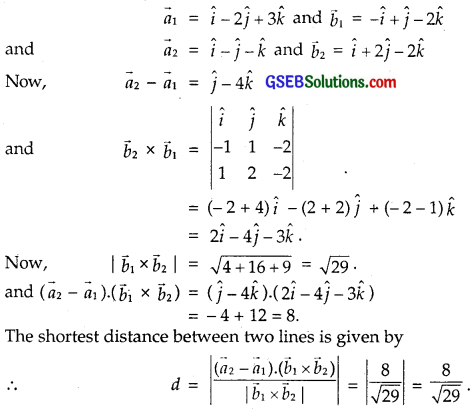# GSEB Solutions Class 12 Maths Chapter 11 Three Dimensional Geometry Ex 11.2

Gujarat Board GSEB Textbook Solutions Class 12 Maths Chapter 11 Three Dimensional Geometry Ex 11.2 Textbook Questions and Answers.

## Gujarat Board Textbook Solutions Class 12 Maths Chapter 11 Three Dimensional Geometry Ex 11.2

Question 1.
Show that the three lines with direction cosines
$$\frac{12}{13}$$, $$\frac{- 3}{13}$$, $$\frac{- 4}{13}$$; $$\frac{4}{13}$$, $$\frac{12}{13}$$, $$\frac{3}{13}$$
and $$\frac{3}{13}$$, $$\frac{- 4}{13}$$, $$\frac{12}{13}$$ are mutually perpendicular.
Solution:
The line with direction cosines l1, m1, n1 and l2, m2, n2 are perpendicular if
l1l2 + m1m2 + n1n2 = 0.

(i) Line with direction cosines $$\frac{12}{13}$$, $$\frac{- 3}{13}$$, $$\frac{- 4}{13}$$ and $$\frac{4}{13}$$, $$\frac{12}{13}$$, $$\frac{3}{13}$$
are perpendicular, if
$$\frac{12}{13}$$ × $$\frac{4}{13}$$ + ($$\frac{- 3}{13}$$)($$\frac{12}{13}$$) + ($$\frac{- 4}{13}$$)($$\frac{3}{13}$$) = 0
or, if $$\frac{48-36-12}{169}$$ = 0, which is true.
⇒ These lines are perpendicular.

(ii) The lines with direction cosines $$\frac{4}{13}$$, $$\frac{12}{13}$$, $$\frac{3}{13}$$ and $$\frac{3}{13}$$, $$\frac{- 4}{13}$$, $$\frac{12}{13}$$
are perpendicular, if
$$\frac{4}{13}$$ × $$\frac{3}{13}$$ + $$\frac{12}{13}$$($$\frac{- 4}{13}$$) + $$\frac{3}{13}$$($$\frac{12}{13}$$) = 0
$$\frac{12-48+36}{169}$$ = 0, which is true.
⇒ These lines are perpendicular.

(iii) the lines with direction cosines $$\frac{3}{13}$$, $$\frac{- 4}{13}$$, $$\frac{12}{13}$$
and $$\frac{12}{13}$$, $$\frac{- 3}{13}$$, $$\frac{- 4}{13}$$ are perpendicular, if
$$\frac{3}{13}$$ × $$\frac{12}{13}$$ + ($$\frac{- 4}{13}$$)($$\frac{- 3}{13}$$) + $$\frac{12}{13}$$ × ($$\frac{- 4}{13}$$)
= $$\frac{36+12-48}{169}$$ = 0, which is true.
∴ These lines are perpendicular.
⇒ Given lines are mutually perpendicular.Question 2.
Show that the line through the points (1, – 1, 2) and (3, 4, – 2) is perpendicular to the line through the points (0, 3, 2) and (3, 5, 6).
Solution:
Let A and B be the points (1, – 1, 2) and (3, 4, – 2) respectively.
Direction ratios of AB are
3 – 1, 4 – (- 1), – 2 – 2 or 2, 5, – 4.
Let C and D be the points (0, 3, 2) and (3, 5, 6) respectively.
Direction ratios of CD are
3 – 0, 5 – 3, 6 – 2 or 3, 2, 4.
AB is perpendicular to CD, if a1a2 + b1b2 + C1C2 = 0.
i.e., 2 × 3 + 5 × 2 + (- 4) × 4
= 6 + 10 – 16 = 0, which is true.
⇒ AB ⊥ CD.

Question 3.
Show that the line through the points (4, 7, 8) and (2, 3, 4) is parallel to the line through the points (- 1, – 2, 1) and (1, 2, 5).
Solution:
Let A and B be the points (4, 7, 8) and (2, 3, 4) respectively.
Direction ratios of AB are 2 – 4, 3 – 7, 4 – 8 or – 2, – 4, – 4 or 1, 2, 2.
Let C and D be the points (- 1, – 2, 1) and (1, 2, 5) respectively.
∴ Direction ratios of CD are
1 – (- 1), 2 – (- 2), 5 – 1 or 2, 4, 4.
AB is parallel to CD if $$\frac{a_{1}}{a_{2}}$$ = $$\frac{b_{1}}{b_{2}}$$ = $$\frac{c_{1}}{c_{2}}$$.
Here, $$\frac{1}{2}$$ = $$\frac{2}{4}$$ = $$\frac{2}{4}$$, which is true.
Hence, AB || CD.Question 4.
Find the equation of the line which passes through the point (1, 2, 3) and is parallel to the vector 3$$\hat {i}$$ + 2$$\hat {j}$$ – 2$$\hat {k}$$.
Solution:
Equation of the line passing through the point a and parallel to vector $$\vec{b}$$ is
$$\vec{r}$$ = $$\vec{a}$$ + λ$$\vec{b}$$
Here, $$\vec{a}$$ = $$\hat {i}$$ + 2$$\hat {j}$$ + 3$$\hat {k}$$ and $$\vec{b}$$ = 3$$\hat {i}$$ + 2$$\hat {j}$$ – 2$$\hat {k}$$.
∴ Equation of the required line is
$$\vec{r}$$ = ($$\hat {i}$$ + 2$$\hat {j}$$ + 3$$\hat {k}$$) + λ(3$$\hat {i}$$ + 2$$\hat {j}$$ – 2$$\hat {k}$$), λ ∈ R.

Question 5.
Find the equation of the line in vector and in cartesian form that passes through the point with position vector 2$$\hat {i}$$ – $$\hat {j}$$ + 4$$\hat {k}$$
and is in the direction $$\hat {i}$$ + 2$$\hat {j}$$ – $$\hat {k}$$.
Solution:
We know that the vector equation of a line passing through a point with position vector $$\vec{a}$$ and parallel to the vector $$\vec{b}$$ is
$$\vec{r}$$ = $$\vec{a}$$ + λ$$\vec{b}$$
Here, $$\vec{a}$$ = 2$$\hat {i}$$ – $$\hat {j}$$ + 4$$\hat {k}$$ and $$\vec{b}$$ = $$\hat {i}$$ + 2$$\hat {j}$$ – $$\hat {k}$$.
So, the vector equation of the required line is
$$\vec{r}$$ = (2$$\hat {i}$$ – $$\hat {j}$$ + 4$$\hat {k}$$) + λ($$\hat {i}$$ + 2$$\hat {j}$$ – $$\hat {k}$$) ……………. (1),
where λ is parameter.

In Cartesiant Form :
Putting $$\vec{r}$$ = x$$\hat {i}$$ + y$$\hat {j}$$ + z$$\hat {k}$$ in (1), we get
x$$\hat {i}$$ + y$$\hat {j}$$ + z$$\hat {k}$$ = (2$$\hat {i}$$ – $$\hat {j}$$ + 4$$\hat {k}$$) + λ($$\hat {i}$$ + 2$$\hat {j}$$ – $$\hat {k}$$)
⇒ x$$\hat {i}$$ + y$$\hat {j}$$ + z$$\hat {k}$$ = (2 + λ)$$\hat {i}$$ + (- 1 + 2λ)$$\hat {j}$$ + (4 – λ)$$\hat {k}$$
Equating coefficients of $$\hat {i}$$, $$\hat {j}$$ and $$\hat {k}$$, we get
x = 2 + λ, y = – 1 + 2λ, z = 4 – λ
⇒ x – 2 = λ, $$\frac{y+1}{2}$$ = λ, $$\frac{z-4}{-1}$$ = λ
Eliminating λ, we have:
$$\frac{x-2}{1}$$ = $$\frac{y+1}{2}$$ = $$\frac{z-4}{-1}$$.

Alternative Method :
The equation (1) represents a line passing through a point (2, – 1, 4) and has direction ratios 1, 2, – 1.
∴ The cartisian form of its equations is
$$\frac{x-2}{1}$$ = $$\frac{y+1}{2}$$ = $$\frac{z-4}{-1}$$.Question 6.
Find the cartesian equation of the line which passes through the point (- 2, 4, – 5) and parallel to the line given by
$$\frac{x+3}{3}$$ = $$\frac{y-4}{5}$$ = $$\frac{z+8}{6}$$.
Solution:
The Cartesian equation of the line passing through the point (- 2, 4, – 5) and parallel to the line
$$\frac{x+3}{3}$$ = $$\frac{y-4}{5}$$ = $$\frac{z+8}{6}$$ is $$\frac{x+2}{3}$$ = $$\frac{y-4}{5}$$ = $$\frac{z+5}{6}$$.

Question 7.
The cartesian equation of a line $$\frac{x-5}{3}$$ = $$\frac{y+4}{7}$$ = $$\frac{z-6}{2}$$. Write its vector form.
Solution:
The Cartesian equation of the line is
$$\frac{x-5}{3}$$ = $$\frac{y+4}{7}$$ = $$\frac{z-6}{2}$$ ……………. (1)
Clearly (1) passes through the point A(5, – 4, 6) and has 3, 7, 2 as its direction ratios
⇒ Line (1) passes through the point A with position vector $$\vec{a}$$ = 5$$\hat {i}$$ – 4$$\hat {j}$$ + 6$$\hat {k}$$
and is in the direction of $$\vec{b}$$ = 3$$\hat {i}$$ + 7$$\hat {j}$$ + 2$$\hat {k}$$.
So, the vector equation of the required line is
$$\vec{r}$$ = (5$$\hat {i}$$ – 4$$\hat {j}$$ + 6$$\hat {k}$$) + λ(3$$\hat {i}$$ + 7$$\hat {j}$$ + 2$$\hat {k}$$).Question 8.
Find the vector and cartesian equations of the line that passes through the origin and the point (5, – 2, 3).
Solution:
The line passes through the origin (0, 0, 0)
∴ $$\vec{a}$$ = $$\vec{0}$$
Direction ratios of the line passing through the points (x1, y1, z1) and (x2, y2, z2)
are x2 – x1, y2 – y1, z2 – z1.
∴ Direction ratios of the line joining the points A(0, 0, 0) and B(5, – 2, 3) are
∴ 5 – 0, – 2 – 0, 3 – 0 or 5, – 2, 3.
∴ $$\vec{b}$$ = 5$$\hat {i}$$ – 2$$\hat {j}$$ + 3$$\hat {k}$$

(i) Equation of line AB in vector form
$$\vec{r}$$ = $$\vec{a}$$ + λ$$\vec{b}$$
= $$\vec{0}$$ + λ(5$$\hat {i}$$ – 2$$\hat {j}$$ + 3$$\hat {k}$$).

(ii) Equation of the line AB in cartesian form is
$$\frac{x-x_{1}}{a}$$ = $$\frac{y-y_{1}}{b}$$ = $$\frac{z-z_{1}}{c}$$,
where a, b, c are the direction ratios of the line,
which passes through (x1, y1, z1).
Directon ratios are 5, – 2, 3 and the line passes through (0, 0, 0).
∴ Required equation of AB
$$\frac{x-0}{5}$$ = $$\frac{y-0}{- 2}$$ = $$\frac{z-0}{3}$$
or $$\frac{x}{5}$$ = $$\frac{y}{- 2}$$ = $$\frac{z}{3}$$.

Question 9.
Find the vector and cartesian equations of the line that passes through the points P(3, – 2, – 5) and Q(3, – 2, 6).
Solution:
The line PQ passes through the point P(3, – 2, – 5).
∴ $$\vec{a}$$ = 3$$\hat {i}$$ – 2$$\hat {j}$$ – 5$$\hat {k}$$
Direction ratios of PQ, where P and Q are (3, – 2, – 5) and (3, – 2, 6), are
x2 – x1, y2 – y1, z2 – z1
i.e., 3 – 3, – 2 – (- 2), 6 – (- 5) or 0, 0, 11
∴ $$\vec{b}$$ = 11$$\hat {k}$$

(i) Equation of line PQ in vector form is
$$\vec{r}$$ = $$\vec{a}$$ + λ$$\vec{b}$$
= (3$$\hat {i}$$ – 2$$\hat {j}$$ – 5$$\hat {k}$$) + λ 11$$\hat {k}$$
or $$\vec{r}$$ = (3$$\hat {i}$$ – 2$$\hat {j}$$ – 5$$\hat {k}$$) + λ 11$$\hat {k}$$

(ii) Equation of the line PQ in cartesian form is
$$\frac{x-x_{1}}{a}$$ = $$\frac{y-y_{1}}{b}$$ = $$\frac{z-z_{1}}{c}$$,
P(x1, y1, z1) is (3, – 2, – 5) and directon ratios a, b, c are 0, 0, 11.
∴ Equation of PQ is
$$\frac{x-3}{0}$$ = $$\frac{y+2}{0}$$ = $$\frac{z+5}{11}$$.Question 10.
Find the angle between the following pairs of lines:
(i) $$\vec{r}$$ = 2$$\hat {i}$$ – 5$$\hat {j}$$ + $$\hat {k}$$ + λ(3$$\hat {i}$$ + 2$$\hat {j}$$ + 6$$\hat {k}$$) and
$$\vec{r}$$ = 7$$\hat {i}$$ – 6$$\hat {k}$$ + λ($$\hat {i}$$ + 2$$\hat {j}$$ + 2$$\hat {k}$$)

(ii) $$\vec{r}$$ = 3$$\hat {i}$$ + $$\hat {j}$$ – 2$$\hat {k}$$ + λ($$\hat {i}$$ – $$\hat {j}$$ – 2$$\hat {k}$$) and
$$\vec{r}$$ = 2$$\hat {i}$$ – $$\hat {j}$$ – 56$$\hat {k}$$ + µ(3$$\hat {i}$$ – 5$$\hat {j}$$ – 4$$\hat {k}$$)
Solution:
(i) Let θ be the angle between the given lines. The given lines are parallel to the vectors
$$\vec{b}_{1}$$ = 3$$\hat {i}$$ + 2$$\hat {j}$$ + 6$$\hat {k}$$ and
$$\vec{b}_{2}$$ = $$\hat {i}$$ + 2$$\hat {j}$$ + 2$$\hat {k}$$ respectively.
∴ The angle θ between them is given by(ii) Let θ be the angle between the given lines. The given lines are parallel to the vectors
$$\vec{b}_{1}$$ = $$\hat {i}$$ – $$\hat {j}$$ – 2$$\hat {k}$$ and
$$\vec{b}_{2}$$ = 3$$\hat {i}$$ – 5$$\hat {j}$$ – 4$$\hat {k}$$ respectively.
∴ The angle θ between them is given by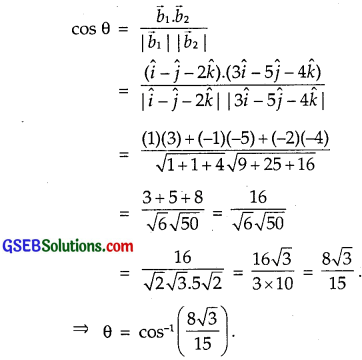Question 11.
Find the angle between the following pair of lines:
(i) $$\frac{x-2}{2}$$ = $$\frac{y-1}{5}$$ = $$\frac{z+3}{-3}$$ and $$\frac{x+2}{-1}$$ = $$\frac{y-4}{8}$$ = $$\frac{z-5}{4}$$.
(ii) $$\frac{x}{2}$$ = $$\frac{y}{2}$$ = $$\frac{z}{1}$$ and $$\frac{x-5}{4}$$ = $$\frac{y-2}{1}$$ = $$\frac{z-3}{8}$$.
Solution:
(i) Let $$\vec{b}_{1}$$ and $$\vec{b}_{2}$$ be the vectors parallel to
$$\frac{x-2}{2}$$ = $$\frac{y-1}{5}$$ = $$\frac{z+3}{-3}$$ and $$\frac{x+2}{-1}$$ = $$\frac{y-4}{8}$$ = $$\frac{z-5}{4}$$ respectively.
∴ $$\vec{b}_{1}$$ = 2$$\hat {i}$$ + 5$$\hat {j}$$ – 3$$\hat {k}$$
and $$\vec{b}_{2}$$ = – $$\hat {i}$$ + 8$$\hat {j}$$ + 4$$\hat {k}$$(ii) The given equations are
$$\frac{x}{2}$$ = $$\frac{y}{2}$$ = $$\frac{z}{1}$$ ……………… (1)
and $$\frac{x-5}{4}$$ = $$\frac{y-2}{1}$$ = $$\frac{z-3}{8}$$ ……………….. (2)
Let $$\vec{b}_{1}$$ and $$\vec{b}_{2}$$ be the vectors parallel to (1) and (2) respectively.
Then, $$\vec{b}_{1}$$ = 2$$\hat {i}$$ + 2$$\hat {j}$$ + $$\hat {k}$$
and $$\vec{b}_{2}$$ = 4$$\hat {i}$$ + $$\hat {j}$$ + 8$$\hat {k}$$.
If θ is the angle between the given lines, then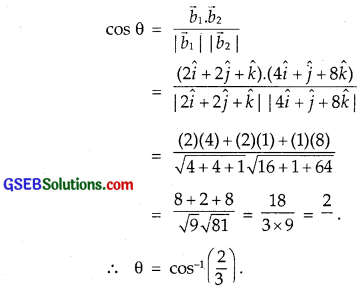Question 12.
Find the value of p so that the lines
$$\frac{1-x}{3}$$ = $$\frac{7y-14}{2p}$$ = $$\frac{z-3}{2}$$ and $$\frac{7-7x}{3p}$$ = $$\frac{y-5}{1}$$ = $$\frac{6-z}{5}$$ are at right angles.
Solution:
The given equations are not in the standard form. The equations of the given lines can be respectively written as,The direction ratios of the given lines are – 3, $$\frac{2p}{7}$$, 2 and $$\frac{-3p}{7}$$, 1, – 5.
The line are perpendicular to each other.Question 13.
Show that the lines $$\frac{x-5}{7}$$ = $$\frac{y+2}{-5}$$ = $$\frac{z}{1}$$
and $$\frac{x}{1}$$ = $$\frac{y}{2}$$ = $$\frac{z}{3}$$ are perpendicular to each other.
Solution:
The given lines are
$$\frac{x-5}{7}$$ = $$\frac{y+2}{-5}$$ = $$\frac{z}{1}$$ …………….. (1)
and $$\frac{x}{1}$$ = $$\frac{y}{2}$$ = $$\frac{z}{3}$$ …………….. (2)
Let $$\vec{b}_{1}$$ = 7$$\hat {i}$$ – 5$$\hat {j}$$ + $$\hat {k}$$
and $$\vec{b}_{2}$$ = $$\hat {i}$$ + 2$$\hat {j}$$ + 3$$\hat {k}$$.
If θ is the angle between the given lines, then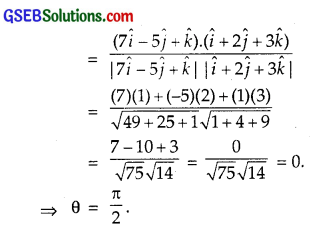Hence, the given lines are perpendicular to each other.Question 14.
Find the shortest distance between the lines
$$\vec{r}$$ = ($$\hat {i}$$ + 2$$\hat {j}$$ + $$\hat {k}$$) + λ($$\hat {i}$$ – $$\hat {j}$$ + $$\hat {k}$$) …………… (1)
and $$\vec{r}$$ = (2$$\hat {i}$$ – $$\hat {j}$$ – $$\hat {k}$$) + µ(2$$\hat {i}$$ + $$\hat {j}$$ + 2$$\hat {k}$$) …………… (2)
The shortest distance between the linesComparing the equations (1) and (2) with $$\vec{r}$$ = $$\vec{a}_{1}$$ + λ$$\vec{b}_{1}$$ and $$\vec{r}$$ = $$\vec{a}_{2}$$ + µ$$\vec{b}_{2}$$ respectively, we have: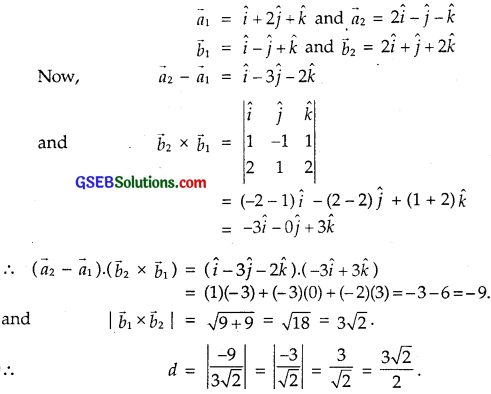Question 15.
Find the shortest distance between the lines
$$\frac{x+1}{7}$$ = $$\frac{y+1}{-6}$$ = $$\frac{z+1}{1}$$ and $$\frac{x-3}{1}$$ = $$\frac{y-5}{-2}$$ = $$\frac{z-7}{1}$$.
Solution:
Shortest distance between the lines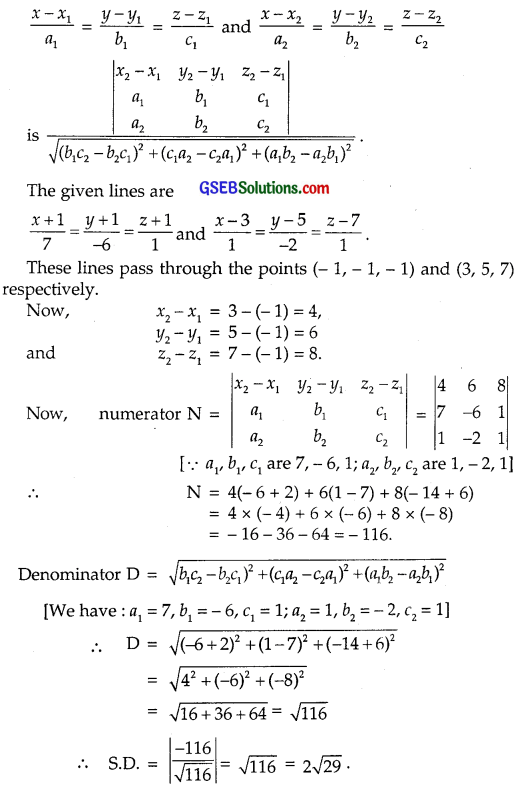Question 16.
Find the shortest distance the lines whose vector equations are
$$\vec{r}$$ = ($$\hat {i}$$ + 2$$\hat {j}$$ + 3$$\hat {k}$$) + λ($$\hat {i}$$ – 3$$\hat {j}$$ + 2$$\hat {k}$$) and …………… (1)
$$\vec{r}$$ = (4$$\hat {i}$$ + 5$$\hat {j}$$ + 6$$\hat {k}$$) +µ(2$$\hat {i}$$ + 3$$\hat {j}$$ + $$\hat {k}$$) …………… (2)
We know that the shortest distance between the lines areComparing the equations (1) and (2) with $$\vec{r}$$ = $$\vec{a}_{1}$$ + λ$$\vec{b}_{1}$$ and
$$\vec{r}$$ = $$\vec{a}_{2}$$ + µ$$\vec{b}_{2}$$ respectively, we have:Question 17.
Find the shortest distance between the lines whose vector equations are
$$\vec{r}$$ = (1 – t)$$\hat {i}$$ + (t – 2)$$\hat {j}$$ + (3 – 2)$$\hat {k}$$ ………………. (1) and
$$\vec{r}$$ = ($$\hat {i}$$ – $$\hat {j}$$ – $$\hat {k}$$) + s($$\hat {i}$$ + 2$$\hat {j}$$ – 2$$\hat {k}$$) …………… (2)
Solution:
The given equations can be written as
$$\vec{r}$$ = $$\hat {i}$$ – 2$$\hat {j}$$ + 3$$\hat {k}$$ + t(- $$\hat {i}$$ + $$\hat {j}$$ – 2$$\hat {k}$$) ………….. (1)
and $$\vec{r}$$ = ($$\hat {i}$$ – $$\hat {j}$$ – $$\hat {k}$$) + s($$\hat {i}$$ + 2$$\hat {j}$$ – 2$$\hat {k}$$) ……………… (2)
Comparing these equations with